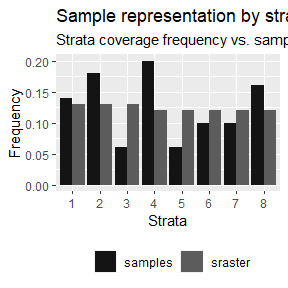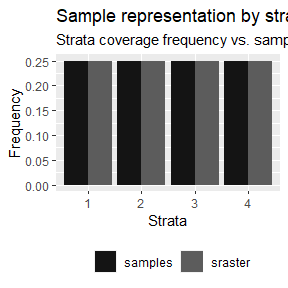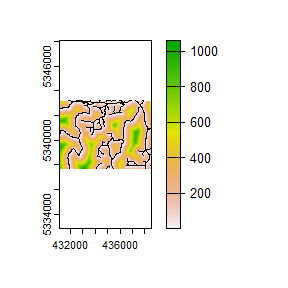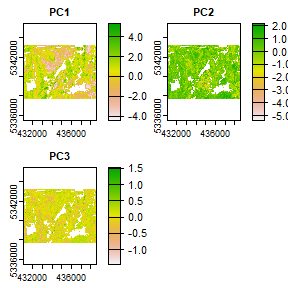# Calculating

Currently, there are 8 functions associated with the calculate verb in the sgsR package:

Algorithm Description
calculate_representation() Determine representation of strata in existing sample unit
calculate_distance() Per pixel distance to closest access vector
calculate_pcomp() Principal components of input mraster
calculate_sampsize() Estimated sample sizes based on relative standard error
calculate_allocation() Proportional / optimal / equal / manual allocation
calculate_coobs() Detail how existing sample units are distributed among mraster
calculate_pop() Population level information (PCA / quantile matrix / covariance matrix) of mraster
calculate_lhsOpt() Determine optimal Latin hypercube sampling parameters including sample size

## calculate_representation()

calculate_representation() function allows the users to verify how a stratification is spatially represented in an existing sample. Result in tabular and graphical (if plot = TRUE) outputs that compare strata coverage frequency and sampling frequency.

#--- quantile sraster ---#
quantiles <- strat_quantiles(mraster = mraster$zq90, nStrata = 8) #--- random samples ---# srs <- sample_srs(raster = sraster, nSamp = 50) #--- calculate representation ---# calculate_representation(sraster = quantiles, existing = srs, plot = TRUE)#> # A tibble: 8 × 6 #> strata srasterFreq sampleFreq diffFreq nSamp need #> <int> <dbl> <dbl> <dbl> <int> <dbl> #> 1 1 0.13 0.14 0.0100 7 0 #> 2 2 0.13 0.18 0.05 9 -2 #> 3 3 0.13 0.06 -0.07 3 4 #> 4 4 0.12 0.2 0.08 10 -4 #> 5 5 0.12 0.06 -0.06 3 3 #> 6 6 0.12 0.1 -0.0200 5 1 #> 7 7 0.12 0.1 -0.0200 5 1 #> 8 8 0.12 0.16 0.04 8 -2 The tabular output presents the frequency of coverage for each strata (srasterFreq) (what % of the landscape does the strata cover) and the sampling frequency within each strata (sampleFreq) (what % of total existing samples are in the strata). The difference (diffFreq) between coverage frequency and sampling frequency determines whether the values are over-represented (positive numbers) or under-represented (negative numbers). This value translates to a discrete need attribute that defines whether there is a need to add or remove samples to meet the number of samples necessary to be considered representative of the spatial coverage of strata inputted in sraster. Performing the algorithm on a sample set derived using sample_strat() exhibits proportional sampling to strata coverage given that samples were allocated proportionally to spatial coverage. calculate_representation(sraster = sraster, existing = existing, plot = TRUE)#> # A tibble: 4 × 6 #> strata srasterFreq sampleFreq diffFreq nSamp need #> <int> <dbl> <dbl> <dbl> <int> <dbl> #> 1 1 0.25 0.25 0 50 0 #> 2 2 0.25 0.25 0 50 0 #> 3 3 0.25 0.25 0 50 0 #> 4 4 0.25 0.25 0 50 0 TIP! Presence of very small (negligible) differences between srasterFreq and sampleFreq is common. In these situations, it is important for the user to determine whether to add or remove the samples. ## calculate_distance calculate_distance() uses input raster and access data and outputs the per pixel distance to the nearest access point. This algorithm has a specific value for constraining sampling protocols, such as with sample_clhs(), where the output raster layer can be used as the cost for the constraint. The output raster consists of the input appended with the calculated distance layer (dist2access). calculate_distance(raster = sraster, # input access = access, # define access road network plot = TRUE) # plot #> |---------|---------|---------|---------| =========================================#> class : SpatRaster #> dimensions : 277, 373, 2 (nrow, ncol, nlyr) #> resolution : 20, 20 (x, y) #> extent : 431100, 438560, 5337700, 5343240 (xmin, xmax, ymin, ymax) #> coord. ref. : UTM Zone 17, Northern Hemisphere #> source(s) : memory #> names : strata, dist2access #> min values : 1, 0.3438 #> max values : 4, 1061.6599 This function performs considerably slower when access has many features. Consider mergeing features for improved efficiency. ## calculate_pcomp calculate_pcomp() uses mraster as the input and performs principal component analysis. The number of components defined by the nComp parameter specifies the number of components that will be rasterized. calculate_pcomp(mraster = mraster, # input nComp = 3, # number of components to output plot = TRUE, # plot details = TRUE) # details about the principal component analysis appended#>$pca
#> Standard deviations (1, .., p=3):
#>  1.5479878 0.7359109 0.2493371
#>
#> Rotation (n x k) = (3 x 3):
#>                PC1        PC2        PC3
#> zq90     0.6286296 -0.1795433 -0.7566961
#> pzabove2 0.5104140  0.8293596  0.2272450
#> zsd      0.5867729 -0.5290812  0.6130014
#>
#> $raster #> class : SpatRaster #> dimensions : 277, 373, 3 (nrow, ncol, nlyr) #> resolution : 20, 20 (x, y) #> extent : 431100, 438560, 5337700, 5343240 (xmin, xmax, ymin, ymax) #> coord. ref. : UTM Zone 17, Northern Hemisphere #> source(s) : memory #> names : PC1, PC2, PC3 #> min values : -4.402269, -5.357801, -1.446156 #> max values : 5.282663, 2.155242, 1.510955 ## calculate_sampsize calculate_sampsize() function allows the user to estimate an appropriate sample size using the relative standard error (rse) of input metrics. If the input mraster contains multiple layers, the sample sizes will be determined for all layers. If plot = TRUE and rse is defined, a sequence of rse values will be visualized with the indicators and the values for the matching sample size. #--- determine sample size based on relative standard error (rse) of 1% ---# calculate_sampsize(mraster = mraster, rse = 0.01) #> nSamp rse var #> 1 1394 0.01 zq90 #> 2 1341 0.01 pzabove2 #> 3 1859 0.01 zsd #--- change default threshold sequence values ---# #--- if increment and rse are not divisible the closest value will be taken ---# p <- calculate_sampsize(mraster = mraster, rse = 0.025, start = 0.01, end = 0.08, increment = 0.01, plot = TRUE) #> 'rse' not perfectly divisible by 'increment'. Selecting closest sample size (rse = 0.03) based on values. p #>$nSamp
#> # A tibble: 3 × 3
#> # Groups:   var 
#>   nSamp   rse var
#>   <dbl> <dbl> <chr>
#> 1   157  0.03 zq90
#> 2   151  0.03 pzabove2
#> 3   211  0.03 zsd
#>

### Optimal Allocation

Sample units are allocated based on within strata variance. The optimal allocation method uses the variation within the strata metric to allocate sample units. This means that in addition to providing an sraster, that a specific metric (mraster) must be provided to calculate variation to optimally allocate sample units.

calculate_allocation(sraster = sraster, # stratified raster
nSamp = 200, # desired sample number
existing = e.sr, #existing samples
allocation = "optim", # optimal allocation
mraster = mraster$zq90, # metric raster force = TRUE) # force nSamp number #> # A tibble: 4 × 3 #> # Rowwise: #> strata total need #> <dbl> <dbl> <dbl> #> 1 1 27 77 #> 2 2 -14 36 #> 3 3 -25 25 #> 4 4 12 63 ### Equal allocation There may be situations where the user wants an equal number of sample units allocated to each strata. In these situations use allocation = equal. In this case, nSamp refers to the total number of sample units per strata, instead of the overall total number. calculate_allocation(sraster = sraster, # stratified raster nSamp = 20, # desired sample number allocation = "equal") # optimal allocation #> Implementing equal allocation of samples. #> # A tibble: 4 × 2 #> strata total #> <dbl> <dbl> #> 1 1 20 #> 2 2 20 #> 3 3 20 #> 4 4 20 The code in the demonstration above yields a total of 80 samples (20 nSamp for each of the 4 strata in sraster). ### Manual allocation The user may wish to manually assign weights to strata. In this case, allocation = manual can be used and weights must be provided as a numeric vector (e.g. weights = c(0.2, 0.2, 0.2, 0.4) where sum(weights) == 1). In this case, nSamp will be allocated based on weights. weights <- c(0.2, 0.2, 0.2, 0.4) calculate_allocation(sraster = sraster, # stratified raster nSamp = 20, # desired sample number allocation = "manual", # manual allocation weights = weights) # weights adding to 1 #> Implementing allocation of samples based on user-defined weights. #> strata total #> 1 1 4 #> 2 2 4 #> 3 3 4 #> 4 4 8 The code above yields a total of 20 sample units with plots being allocated based on the weights provided in ascending strata order. ## Sample evaluation algorithms The following algorithms were initially developed by Dr. Brendan Malone from the University of Sydney. Dr. Malone and his colleagues supplied an in depth description of the functionality of these algorithms, which were originally developed to improve soil sampling strategies. These functions were modified and implemented to be used for structurally guided sampling approaches. Many thanks to Dr. Malone for being a proponent of open source algorithms. Please consult the original reference for these scripts and ideas as their publication holds helpful and valuable information to understand their rationale for sampling and algorithm development. Malone BP, Minansy B, Brungard C. 2019. Some methods to improve the utility of conditioned Latin hypercube sampling. PeerJ 7:e6451 DOI 10.7717/peerj.6451 ### calculate_coobs calculate_coobs() function performs the COunt of OBServations (coobs) algorithm using an existing sample and mraster. This algorithm helps the user understand how an existing sample is distributed among the landscape in relation to mraster data. TIP! The output coobs raster can be used to constrain clhs sampling using the sample_clhs() function to the areas that are under-represented. The coobs raster determines how many observations are similar in terms of the covariate space at every pixel, and takes advantage of parallel processing routines. calculate_coobs(mraster = mraster, # input existing = existing, # existing samples cores = 4, # parallel cores to use details = TRUE, # provide details from algorithm output plot = TRUE) # plot ## Latin hypercube sampling evaluation algorithms The following 2 algorithms present the means to maximize the effectiveness of the latin hypercube sampling protocols. ### calculate_pop calculate_pop() calculates population level statistics of an mraster, including calculating the principal components, quantile & covariate distributions, and Kullback-Leibler divergence testing. The outputs produced from this functions are required to use the calculate_lhsOpt() function described in the following section. TIP! This algorithm can be pre-emptively used to calculate matQ and MatCov, two values that are used for the sample_ahels() function. This will save processing time during sampling. #--- by default all statistical data are calculated ---# calculate_pop(mraster = mraster) # input  The output list contains the following: • $values - Pixel values from mraster

• $pcaLoad - PCA loadings • $matQ - Quantile matrix

• \$matCov - Covariate matrix

#--- statistical analyses can be chosen by setting their parameter to FALSE ---#
mat <- calculate_pop(mraster = mraster, # input
nQuant = 10) # desired number of quantiles

#--- use matrix output within sample ahels algorithm ---#
sample_ahels(mraster = mraster, # input
existing = existing, # existing sample
nQuant = 10, # number of desired quantiles
nSamp = 50, # desired sample size
matCov = mat) # covariance matrix

### calculate_lhsOpt

calculate_lhsOpt() function performs a bootstrapped latin hypercube sampling approach where population level analysis of mraster data is performed to determine the optimal latin hypercube sample size.

calculate_pop() and varying sample sizes defined by minSamp, maxSamp, step and rep. Sampling protocols are conducted and statistical effectiveness of those sampling outcomes are evaluated to determine where sample size is minimized and statistical representation is maximized.

#--- calculate lhsPop details ---#
poplhs <- calculate_pop(mraster = mr)

calculate_lhsOpt(popLHS = poplhs)
calculate_lhsOpt(popLHS = poplhs,
PCA = FALSE,
iter = 200)